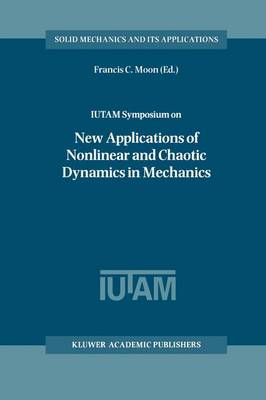•# Guaranteed Accuracy in Numerical Linear Algebra - Mathematics and Its Applications 252 (Hardback)

(author), (author), (author), (author)
£114.00
Hardback 537 Pages
Published: 30/06/1993
• We can order this from the publisher

UK delivery within 3-4 weeks

There exists a vast literature on numerical methods of linear algebra. In our bibliography list, which is by far not complete, we included some monographs on the subject , , , , , . The present book is devoted to the theory of algorithms for a single problem of linear algebra, namely, for the problem of solving systems of linear equations with non-full-rank matrix of coefficients. The solution of this problem splits into many steps, the detailed discussion of which are interest ing problems on their own (bidiagonalization of matrices, computation of singular values and eigenvalues, procedures of deflation of singular values, etc. ). Moreover, the theory of algorithms for solutions of the symmetric eigenvalues problem is closely related to the theory of solv ing linear systems (Householder's algorithms of bidiagonalization and tridiagonalization, eigenvalues and singular values, etc. ). It should be stressed that in this book we discuss algorithms which to computer programs having the virtue that the accuracy of com lead putations is guaranteed. As far as the final program product is con cerned, this means that the user always finds an unambiguous solution of his problem. This solution might be of two kinds: 1. Solution of the problem with an estimate of errors, where abso lutely all errors of input data and machine round-offs are taken into account. 2.

Publisher: Springer
ISBN: 9780792323525
Number of pages: 537
Weight: 2100 g
Dimensions: 235 x 155 x 30 mm
Edition: 1993 ed.